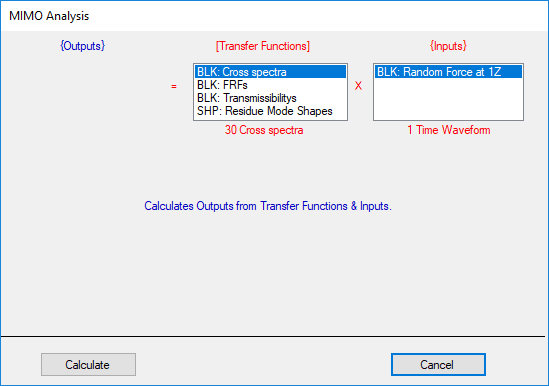# Transform | MIMO Outputs

Calculates multiple Outputs from Transfer Functions & Inputs.  Different types of Inputs can be used for this calculation,

• Input time waveforms.

• Input Auto spectra or Fourier spectra.

Before using this command,

• All Inputs must be designated as Input (or Both) in the Input Output column of the M#s spreadsheet.

• All Transfer Functions must be designated as Cross in the Input Output column of the M#s spreadsheet

• Residue mode shapes or UMM mode shapes can also be used to synthesize the required FRFs

When this command is executed, the following dialog box is opened,• The Data Block containing the Inputs should be chosen from the Inputs list box.

• The Data Block containing the Transfer Functions (or Cross measurements) should be chosen from the Transfer Functions list box.

## Input Time Waveforms

Time waveforms can be imported or synthesized using File | New | Data Block in the ME'scope window.

Input time waveforms are transformed to Fourier spectra before being multiplied by the Transfer Functions to yield Output Fourier spectra.
The Output Fourier spectra are then transformed to time waveforms.

## FRFs from Modal Parameters

FRFs can be imported, acquired with an Acquisition window, or synthesized from a modal model .

• If a Shape Table with modal parameters in it is chosen instead of a Transfer Function Data Block, the required FRFs are synthesized from the modal parameters.

• The frequency axis parameters of the Input Fourier spectra are used for the FRF synthesis.

• If Residue mode shapes are used to synthesize FRFs, FRFs can only be synthesized with the same DOFs as the Residue mode shapes

• If UMM mode shapes are used to synthesize FRFs, FRFs are synthesized with Reference DOFs to match the DOFs of the Inputs.

## Transfer Function Matrix

Each Transfer Functions is assembled into a Transfer Function matrix based on its Output & Input DOFs.

• The Output DOF designates the row position and the Input DOF designates the column position of each Transfer Function in the Transfer Function matrix.

• The DOF of each Input must match with an Input DOF (column) of the Transfer Function matrix.

• Each calculated Output is given the Output DOF (row) of the Transfer Function matrix.